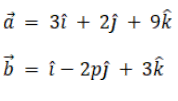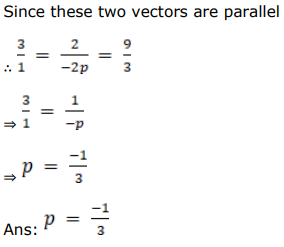# Find the value of p for which the vectorsQuestion:

Find the value of $p$ for which the vectors $\vec{a}=(3 \hat{i}+2 \hat{j}+9 \hat{k})$ and $\overrightarrow{\mathrm{b}}=(\hat{\mathrm{i}}-2 p \hat{\mathrm{j}}+3 \hat{\mathrm{k}})$ are parallel.

Solution: